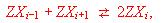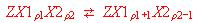Particle-Distribution in a Microporous Material

Andreas Kunzmann, Roland Seifert and Gion Calzaferri
Department of Chemistry and Biochemistry, University of Bern, Freiestrasse 3, CH-3000 Bern 9, Switzerland

Abstract

Equilibria in zeolites and other microporous materials are discussed. We present an independent particles in a box approach, which leads to a thermodynamic description of internal occupation equilibria of the typewhere Z denotes the framework of the material and X the particles that can interchange places. The independent particles in a box are defined by considering a crystal consisting of a finite number of unit cells or boxes each of which can be filled with a specific number of particles. All empty sites in a box have equal probability to be occupied, independent of the number of particles present, as long as sites are available. Each time a particle does fall in a box, the probability for a next one to hit this box is reduced by one divided by the number of sites available in an empty box. Hence, as soon as a box is filled, the probability for a particle to hit it becomes zero. The maximum number of particles in the system is equal to the maximum number of sites in a box, multiplied by the number of boxes. This allows equilibrium constants and the decrease of entropy as a function of the equivalent fraction of exchanging species to be calculated. We show that the plot of the logarithm of the equilibrium constant versus the equivalent fraction of exchanging species is not linear, and that the non-linearity is caused by the decrease of entropy. Based on this observation we suggest the independent particles in a box to be used as a reference for "ideal behaviour" and to serve as a reference for determining activity coefficients. The generalisation of the theory leads to the independent particles in boxes with different sites. It is discussed in detail with regard to two non-equivalent sites corresponding to the internal equilibriain which X1 and X2 are the same species but occupy site 1 and 2, respectively, of a box. We show the solution of this problem and explain the distribution of the particles among the different sites, as a function of the average exchange degree.